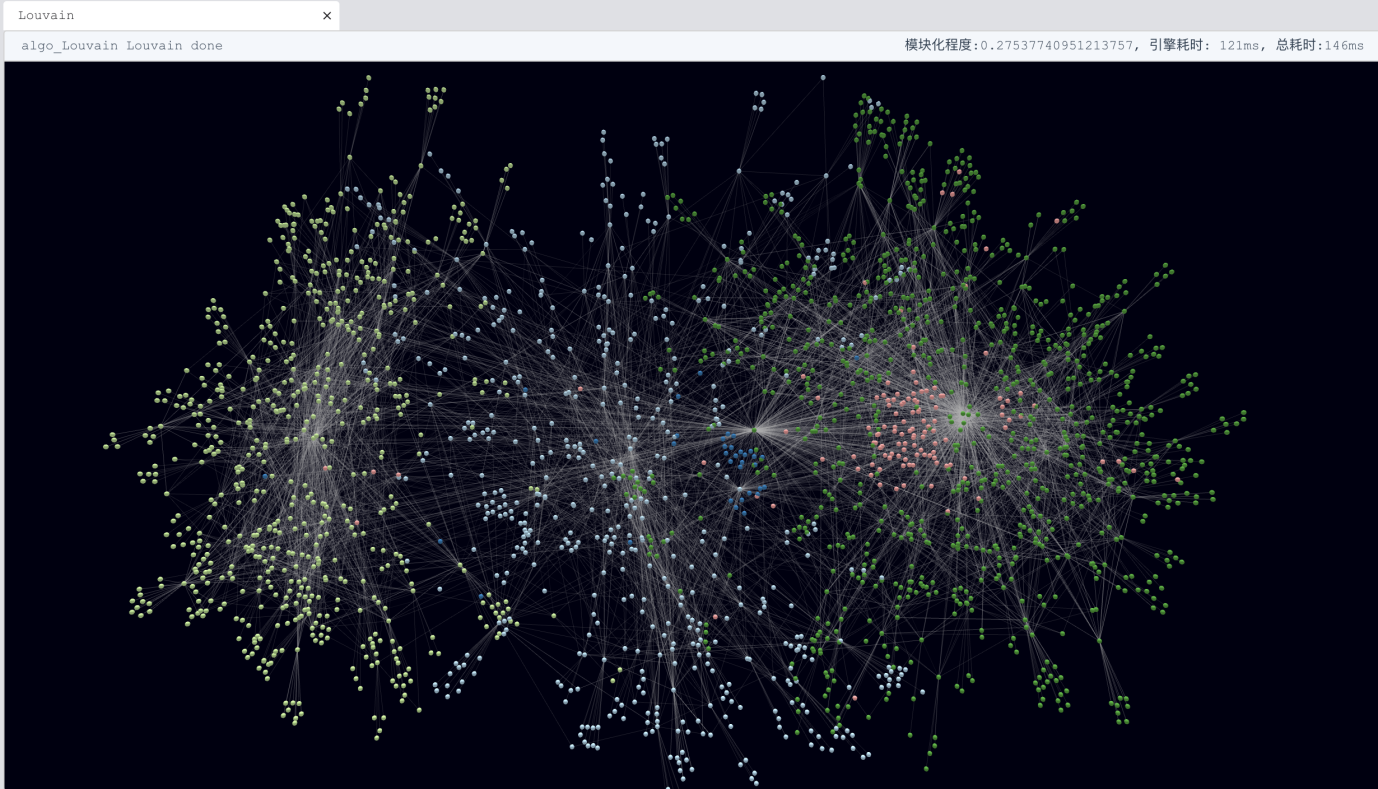# Change Nickname

Current Nickname:
Search
v2.x
v2.x

# Community Detection & Propagation Algorithm

### `algo(page_rank)`

Basic

Page Rank is a way of measuring the importance of nodes in a DIRECTED graph, by allocating and collecting rank via edges. Page Rank by Ultpa Graph is an iterative process initiated by assigning each node with an initial rank of value 1 (can differ among nodes in case of a customized product); in each iteration, the rank of each node is equally divided among, and distributed to its neighbors through the node's out-pointing edges, after which each node aggregates the received ranks via in-pointing edges as its new rank: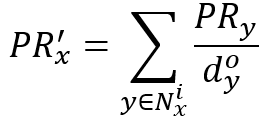Where, PR' is the new rank calculated from PR; N represents the in-pointing-neighbor-set of the subject node x, and d is the out-pointing degree of y, a node from N.

The above function will give a zero result for nodes whose N is empty, so a damping factor q is employed to give those nodes having no in-pointing edges a rank of 1-q, which means a chance of 1-q to be accessed by other nodes: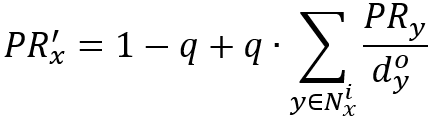The iteration ends when the rank of each node converges individually, or when the iteration limit has been reached. Page Rank by Ultipa Graph is invoked as a task and runs against the entire graph.

Essentially, Page Rank is a link analysis algorithm based on the idea that the importance of a web page depends on the importance of those web pages that believe it is important. Page Rank was originally used by Google to rank web pages in their search engine results, being named after Google's co-founder Larry Page, while coincidently page may stand for 'web pages'. Page Rank is regularly used in bibliometrics, social and information network analysis, and link prediction and recommendation, as well as road networks, biology, chemistry, neuroscience and physics.

Configuration items for Page Rank operation:

Item Data Type Specification Description
`<init_value>` float / (Optional) Inital rank, 0.2 if not configured
`<loop_num>` int >0 The number of loops or iterations
`<damping>` float (0, 1) Damping factor q, usually 0.85; the greater the value, the less rank assigned to nodes with no in-pointing edge
`<limit>` int >0; -1 The maximum number of results to return; -1: return all the results
`<order>` string 'ASC' or 'DESC' (Optional) To arrange the results in ascending or descending order, or leave them un-ordered if not configured

Calculation results:

Item Data Type Range
the actual number of loops int >0
the rank of each node float [0, n], n is the total number of nodes in the graph

Validity of `write_back()`:

Approach Destination
To database node property `#page_rank`
To disk file RPC port

Example 1: To execute Page Rank, setting loop of 5 times, and damping factor of 0.8, write_back 5 records:

``````algo(page_rank)
.params({ loop_num: 5, damping: 0.8, limit: 5 })
.write_back()
``````

### `algo(sybil_rank)`

Sybil Rank is a way to identify fake and malicious accounts (called Sybils) in the OSN (Online Social Networks). It treats the network as an UNDIRECTED graph, propagates trust ranks originated from trusted seeds (non-Sybils)

Sybil Rank is based on the assumption that Sybils tend to have disproportionately limited social connections to non-Sybils (called trusted seeds in a graph), thus would be very slow/late in recieveing trust ranks propagated from those trusted seeds.

Sybil stands for that can mount attacks against real users .

Sybil Rank

Configuration items for Sybil Rank operation:

Item Data Type Specification Description
`<loop_num>` int >0 The number of loops or iterations
`<sybil_num>` int >0 The minimal number of sybils (nodes) to return
`<trust_seeds>` []int Ultipa ID The trusted nodes to start with
`<total_trust>` int >0 The maximal number of trusted nodes

``````algo(sybil_rank).params({
loop_num: 2,
sybil_num: 10,
trust_seeds: [63342,12324,52356,18974,9634,34,8076,…],
total_trust: 100
})
``````

### `algo(lpa)`

Basic

LPA, short for Label Propagation Algorithm, is an iterative process against the entire graph during which a label (value of a particular property) of a node is updated with a neighbor's label that has the largest weight (or highest frequency if no weight applied). The iteration stops when the label of each node converges individually, or when the iteration limit has been reached.

`'Neighbor' here means each node reachable via an edge of the subject node, namely those duplicated neighbors via multi-edges are counted, and so is the subject node itself which needs to multiply by a double number of self-loops it has.`

A neighbor's label weight is the product of the neighbor's weight and the weight of edge connecting it with the subject node: lw = nw · ew. Group these label weights by label values and then aggregate the weights, elect the label value corresponding to the maximum aggregated weight as the new label for the subject node: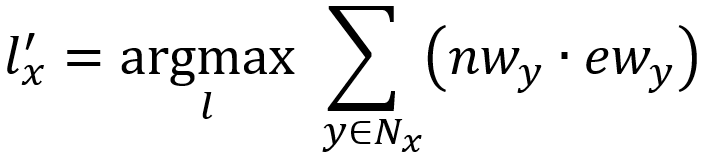Where, l' is the new label value of subject node x elected from l; N is the per-edge-neighbor-set of x, the number of nodes in N equals the degree of x.

At the end of LPA, nodes with the same label are considered in the same community. LPA is widely adopted in many practical scenarios of community detection, such as virtual community mining, multi-media information categorization, etc.

Configuration items for LPA operation:

Item Data Type Specification Description
`<loop_num>` int >0 The number of loops or iterations
`<node_property_name>` string node property (Optional) The node property (must be LTE first) to be used as label, or use node _id as label
`<node_weight_name>` string node property (numberic type) (Optional) The node property (must be LTE first) to be used as weight factor, or use 1 as weight factor if not configured
`<edge_weight_name>` string edge property (numberic type) (Optional) The edge property (must be LTE first) to be used as weight factor, or use 1 as weight factor if not configured

Calculation results:

Item Data Type Range
the actual number of loops int >0
the number of communities int >0
the label of each node / /

Validity of `write_back()`:

Approach Destination
To database node property `#lpa_<label>`
To disk file /

Example 1: Launch LPA, set loop number to be 5, and use value of 'type' as label:

``````algo(lpa)
.params({ loop_num: 5, node_property_name: "type" })
``````

### `algo(hanp)`

HANP, short for Hop Attenuation & Node Preference, is an improved version of LPA that takes into account the degree of neighbor and the vitality of label when calculating the label weight. The new label elected for a subject node x in each iteration by HANP is: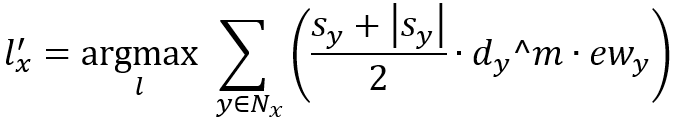Where, s is the label score, and (s+|s|)/2 allows only neighbors with positive scores to participate in the election; d is the degree of neighbor with m being the exponent whose sign suggests whether the label from a neighbor with bigger degree is prefered; ew is the weight of edge connecting neighbor y with the subject node x.

HANP by Ultipa sets the initial label score of each node to 1 (can differ among nodes in case of a customized product); in each iteration the highest score of neighbors that won in the election is attenuated and set as the new score for the subject node: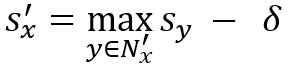Where, N' are the neighbors with the elected label; the attenuation factor δ tells how many times a label can spread (with 1 being the initial score a label can spread 1/δ at most). There are algorithms making effort in extending label's life by subtracting δ only when the new label l' differs from the current label of the subject node.

HANP is a fairly recent invention from the Computer Labs of University of Cambridge (2009), and is supposedly more reliable and computationally efficient in community discovery.

Configuration items for HANP operation:

Item Data Type Specification Description
`<loop_num>` int >0 The number of loops or iterations
`<node_property_name>` string node property The node property (must be LTE first) to be used as label
`<edge_property_name>` string edge property (numeric type) The edge property (must be LTE first) to be used as weight factor
`<m>` float / Preference for neighbor's degree;
m>0: the bigger degree the more preference
m<0: the smaller degree the more preference
m=0: ignore the neighbor's degree
`<delta>` float >=0 The hop attenuation δ that how much a label attenuates each time it spreads

Calculation results:

Item Data Type Range
the actual number of loops int >0
the number of communities int >0
the label of each node / /

Validity of `write_back()`:

Approach Destination
To database node property `#hanp_<label>`
To disk file /

Example 1: Launch HANP, set loop number to be 5, and use value of 'type' as label, 'rating' as edge weight, nodes with less degrees spread easier, and each label spreads maximum 5 times:

``````algo(hanp)
.params({ loop_num: 5, node_property_name: "type", edge_property_name: "rating", m: -1, delta: 0.2})
``````

### `algo(louvain)`

Louvain Community Detection, invented at the University of Louvain in 2008, is a modularity-based community detection algorithm that discovers high grouping of nodes through a method of modularity optimization (or maximization, to be precise) as a fact that modularity of 0.92 suggests a community division significantly better than that with modularity of 0.75.

Modularity is a scale value that measures the relative density of edges inside communities with respect to edges between communities. Modularity of an undirected graph can be defined as: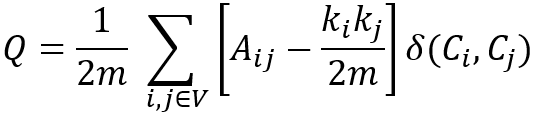Where, i and j are any two nodes from a graph with n nodes and form a number of n * n combinations; when i and j represent different nodes, A is the sum of weights of edges between i and j, when i and j are identical, A is the doubled sum of weights of self-loops of this node, as a loop is considered two edges; k is the weighted degree of a node calculated as the sum of weights of all its edges (with self-loops being doubled); m is the halved sum of weighted degree of all nodes in the graph; C is the community to which a node is assigned, and δ is a dummy variable that takes the value 1 if i and j belong to the same community and otherwise 0.

For nodes from a particular community C, the value of Σ(ki·kj) is equal to (Σki)·(Σkj) that further becomes (Σki)·(Σki), where Σki is the sum of weighted degree of all nodes of community C, called the weighted degree of a community and denoted by Σtot; the value of ΣAij equals Σtot subtract the summed weights of those edges connecting nodes in the community with those outside, which is called the inner weighted degree of a community and denoted by Σin. Re-write the expression of Q with Σtot and Σin: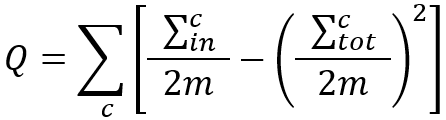So if a node i transfers from community a to communit b, the gain of modularity Q is calculated as: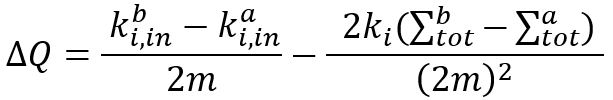Where, Σtot of a and b are calculated without node i, and k(i,in) is the doubled sum of weights of edges between node i and a (or b). This ΔQ helps quickyly identify whether the transfer of a node between two communities will improve the modularity of the current community division.

For the situation that i was the only node of community a, meaning that i formed a community by itself before it transfers, ΔQ is simplified as below: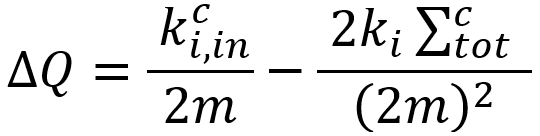Louvain is a composite iterative process with two phases:

Phase1 is a bunch of iterations at the beginning of which each node is considered as a separate community by itself. During an iteration each node tries to find a community of its neighbors to which if it transfers will give a maximum positive ΔQ to the current modularity; each node who has found such a community will be transferred to it at the end of the iteration. Phase1 iterates until none of the nodes can move or when the iteration limit has been reached, and then Phase2 starts.

Phase2 'redraws' the graph by compacting each community from Phase1 as a new node, aggregating all the edges inside a community as a self-loop of the respective new node with a weight of (Σin)/2 of this community, and aggregating the edges between two communities as an edge between the two new nodes with the aggregated weight. If this new structure of node-n-edge is identical with the initial graph structure of this composite, the modularity cannot be maximized further and the algorithm stops; otherwise use this new structure as the initial structure for the next composite of Phase1&2.

`Note: as a way of verification, the calculation of <i>m</i> should stay the same throughout the algorithm, and the modularity <i>Q</i> also stays unchanged before and after Phase2.`

Configuration items for Louvain operation:

Item Data Type Specification Description
`<phase1_loop_num>` int >0 The number of loops or iterations in Phase1 of Louvain, starting from 1 and grow incrementally
`<min_modularity_increase>` float >0 The minimum threshold of ΔQ below which two modularities are considered identical, start from 0.01 or 0.001
`<edge_property_name>` string edge property (numeric type) (Optional) The edge property (must be LTE first) to be used as weight factor, and will use 1 as weight factor if not configured

Calculation results:

Item Data Type Range
the modularity float [-1, 1]
the number of communities int >0
community id of each node int >0

Validity of `write_back()`:

Approach Destination
To database node property `#louvain`
To disk file PRC port

`Note: Ultipa Graph has greatly optimized Louvain to run in a highly efficient and highly parallel way so that even on graphs sized over 10M nodes and edges, it can still be finished in real-time. Oftentimes, we are 10s of thousands of times (or at least hundreds of times) faster than Python or other graph computing frameworks.`

Example: Run Louvain, set loops = 5, ΔQ threshold = 0.01, and use 'ratio' as weight:

``````algo(louvain).params({
phase1_loop_num: 5,
min_modularity_increase: 0.01,
edge_property_name: 'ratio'
})
``````

Below is the screenshot taken from Ultipa Manager on running Louvain with ease. Note that the 4th option is to support real-time Louvain DV (visualization):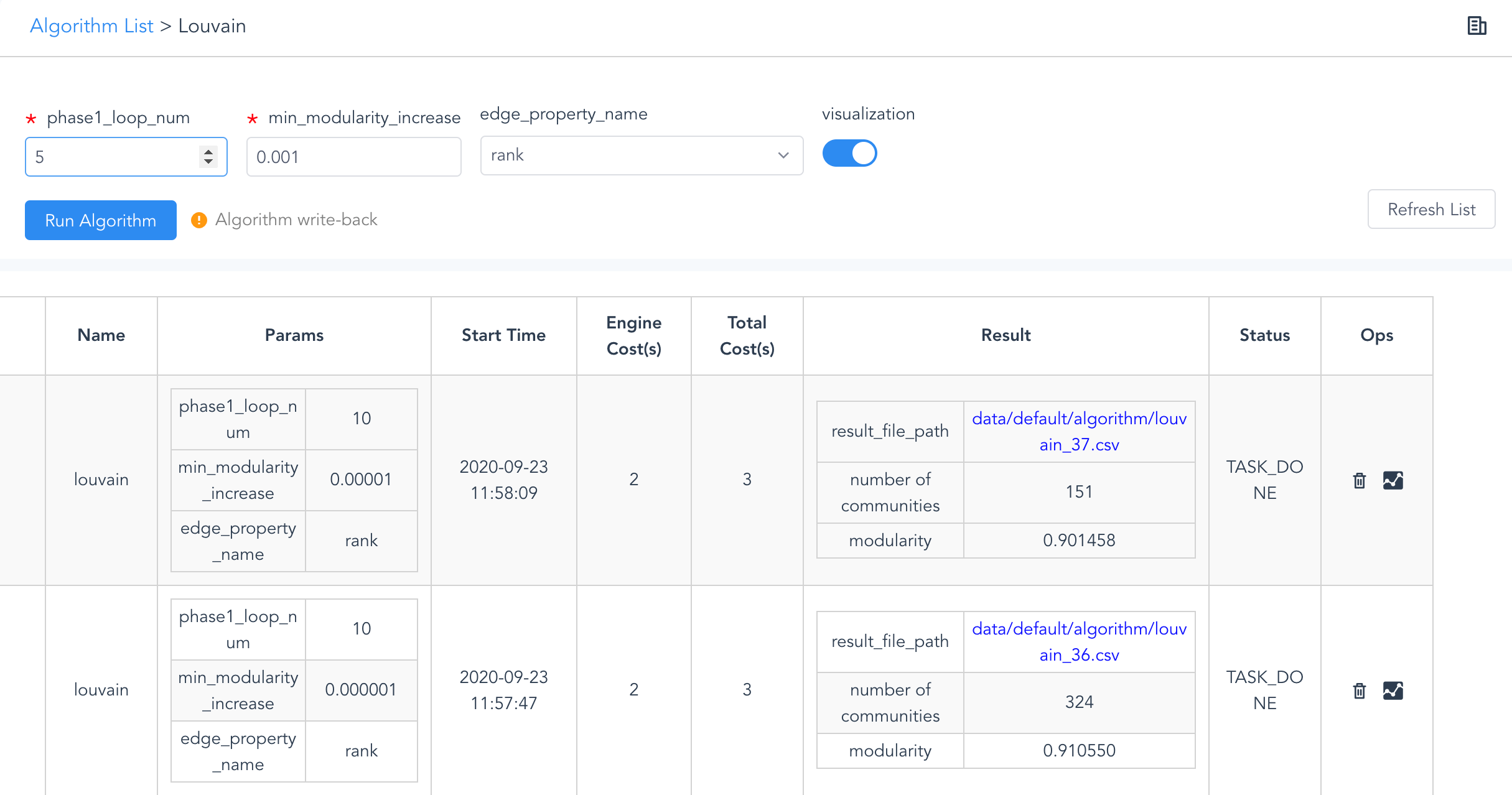Louvain with Visualization (Ultipa Manager)

### `algo_dv(louvain)`

Real-time

Louvain DV is a combinational visualization process that converts the community division calculated by a Louvain operation into the nodes and edges sampled for a visual display, as for example, in the Visual module in Ultipa Manager.

For larger datasets, Louvain DV allows users to sample up to 10,000 nodes for real-time visualization (3D or 2D, accelerated with WebGL); for smaller datasets, the entire data set can be visualized directly.

Configuration items for Louvain DV operation:

Item Data Type Specification Description
`<top>` int (0,10] Top N (number of nodes) communities to display
`<total>` int (0,10000] The nubmer of nodes to sample

Calculation results:

Item Data Type Range
id of edge samples to be displayed int Ultipa ID
id of node samples to be displayed int Ultipa ID
community id of each node int >0

Step 1: Acquire Louvain result for Louvain DV:

``````algo(louvain)
.params({ phase1_loop_num: 5, min_modularity_increase: 0.01 })
.visualization()
``````

Note: The `visualization()` is mandatory in this step. Please check related information in the first chapter.

Step 2: Acquire visualization sample data:

``````algo_dv(louvain)
.params({top: 3, total: 5000})
Note: When invoking algo_dv(), always make sure the algorithm name is the same as the task name, and the task had been run with `.visualization()`.